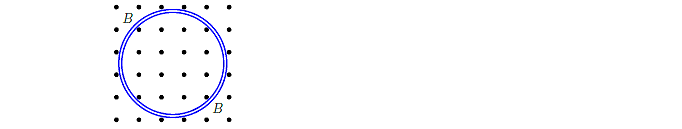🤓 Based on our data, we think this question is relevant for Professor Samarbakhsh's class at LONESTAR.

# Solution: Consider the circular motion of a positively charged particle in the plane of this paper, due to a constant magnetic field B which points out of the paper. Neglect the effect due to gravity. What is the direction of the orbital motion of the particle? Suppose the tangential speed of the charged particle is increased to twice the original tangential speed. (vnew = 2 vold). B is fixed. What is the ratio of the new frequency to the old frequency? Note: Unit for frequency is the number of revolutions per unit time. 1. fnew / fold = 1/3 2. fnew / fold = 2 3. fnew / fold = 1 4. fnew / fold = 3 5. fnew / fold = 1/4 6. fnew / fold = 1/2 7. fnew / fold = 4

###### Problem

Consider the circular motion of a positively charged particle in the plane of this paper, due to a constant magnetic field B which points out of the paper. Neglect the effect due to gravity. What is the direction of the orbital motion of the particle?

Suppose the tangential speed of the charged particle is increased to twice the original tangential speed. (vnew = 2 vold). B is fixed. What is the ratio of the new frequency to the old frequency? Note: Unit for frequency is the number of revolutions per unit time.

1. fnew / fold = 1/3

2. fnew / fold = 2

3. fnew / fold = 1

4. fnew / fold = 3

5. fnew / fold = 1/4

6. fnew / fold = 1/2

7. fnew / fold = 4